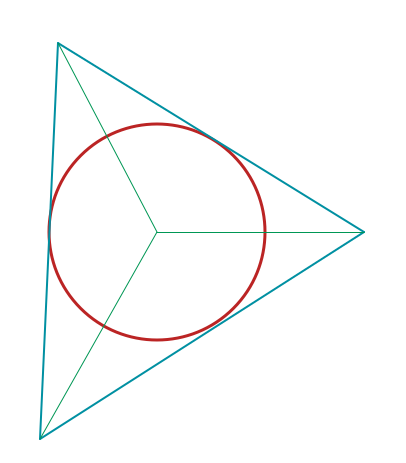# Not enough information

Geometry Level 3A triangle has an area of $\dfrac{43}{10} cm^2$ and its inradius has a length of $\dfrac{4}{5} cm$. Find its perimeter in $cm$. Also, if you can, find the relation between those three variables.

×